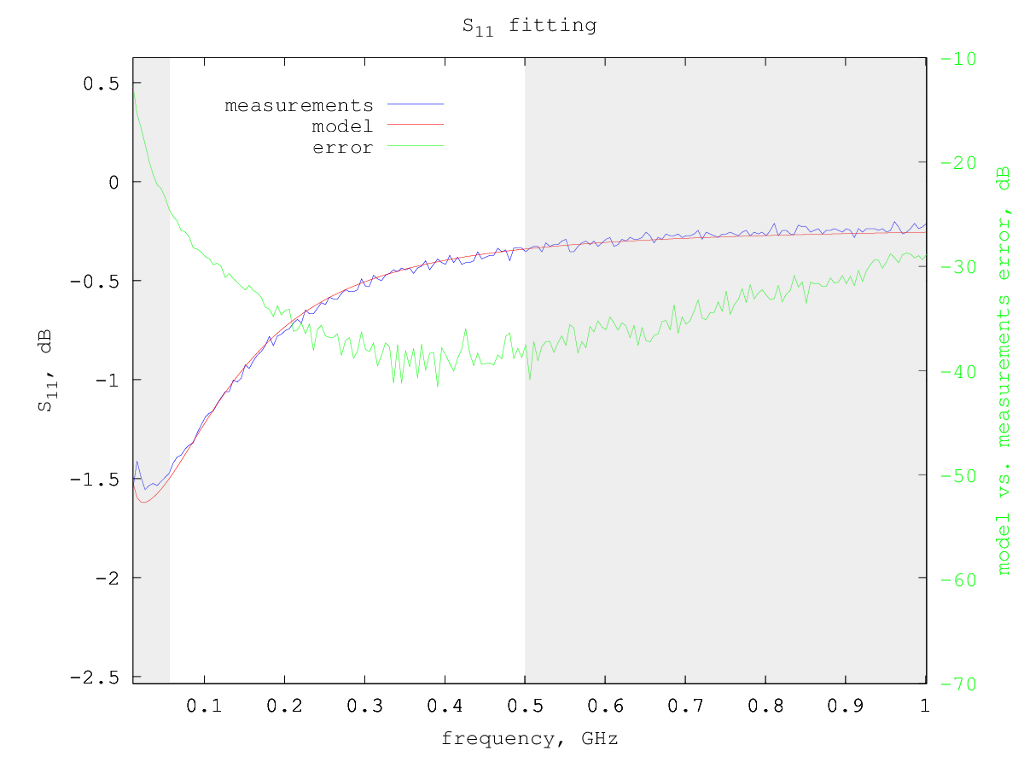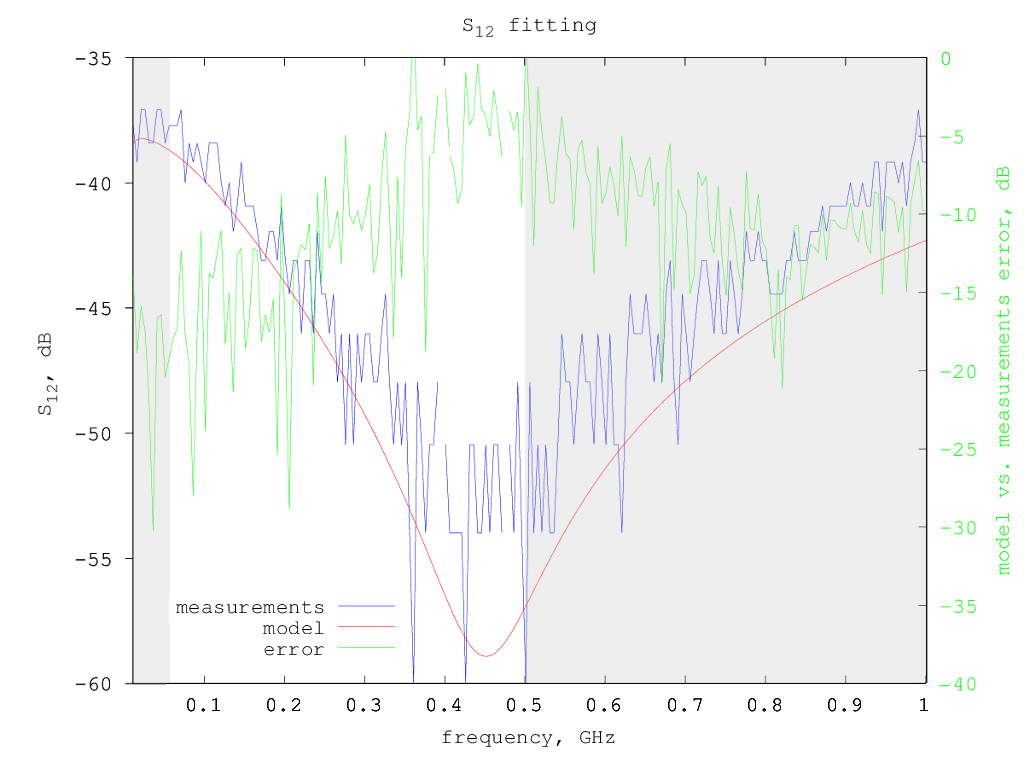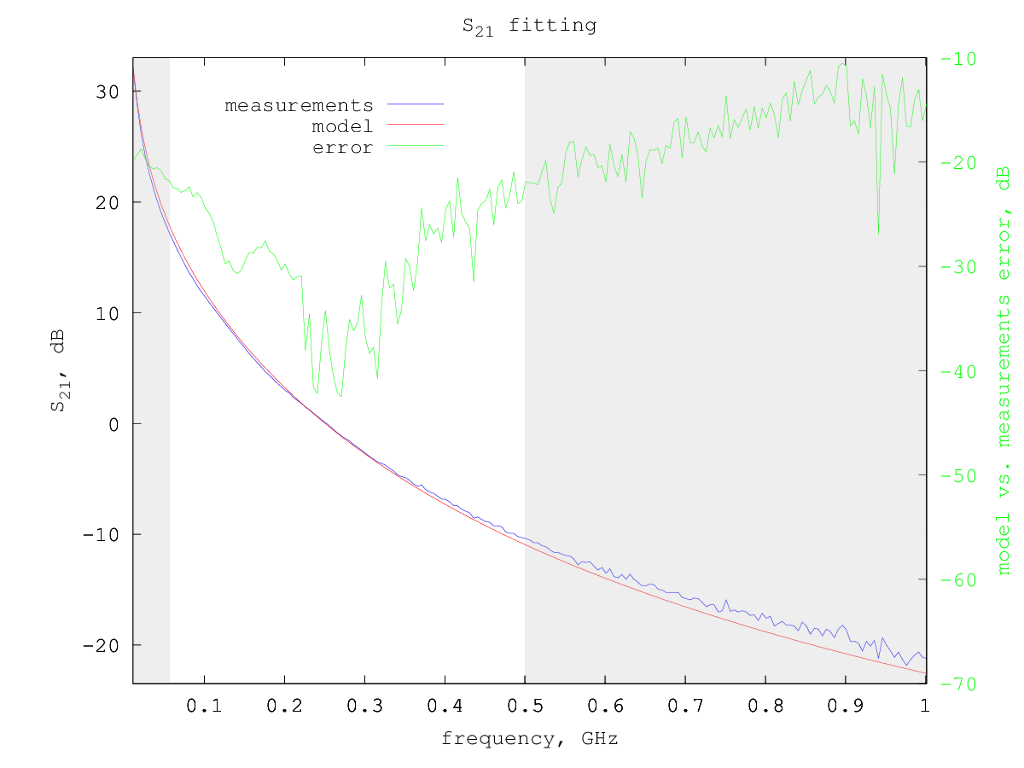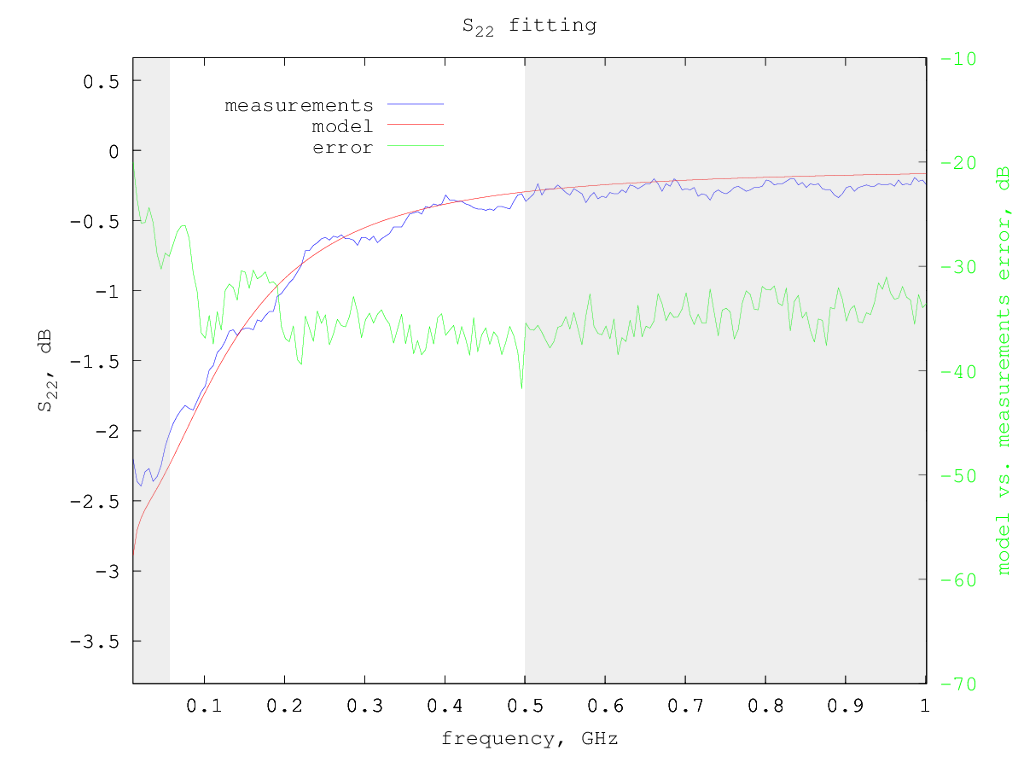# Mitsubishi RD70HHF1 LDMOS model

Under construction...

The Mitsubishi RD70HHF1 is a high-power LDMOS often used in the final stage of commercial HF radios.

# Model for Vdd=12.5 V and Idd= 800 mA

Typical S-parameters for this device can be found on the Mitsubishi web site, for a supply voltage of 12.5 V and a drain current of 800 mA.

Using the extraction method previously described, considering only the S-parameters from 50 MHz to 500 MHz, the following small-signal model parameters are determined:

```Rg = 0.650 ohm
Rd = 0.347 ohm
Rs = 0.0 ohm
Lg = 0.462 nH
Ld = 0.573 nH
Ls = 0.039 nH
Cgs = 193 pF
Cds = 138 pF
Cgd = 9.43 pF
gm = 2.22 S
gds = 11 mS
```
So the equivalent small-signal circuit for this LDMOS at 800 mA drain current is the following:Smith chart with measured vs. model S-parameters:S-parameters fitting details - fitted region is in white background:S12 fit seems poor, but this is mainly due to its low value and noisy measured data: